# Litres to millilitres

Litres to millilitres
Go back to  'Measurement'

Have you ever wondered why some cultures think it is important to have a last name?

It shows their identity and defines their relationship with their forefathers or ancestors.

Similarly, we associate quantities of certain items with their units.

For example, length has meter, volume has litre, time has seconds, and so on.

In this chapter, we will be converting litres to millilitres.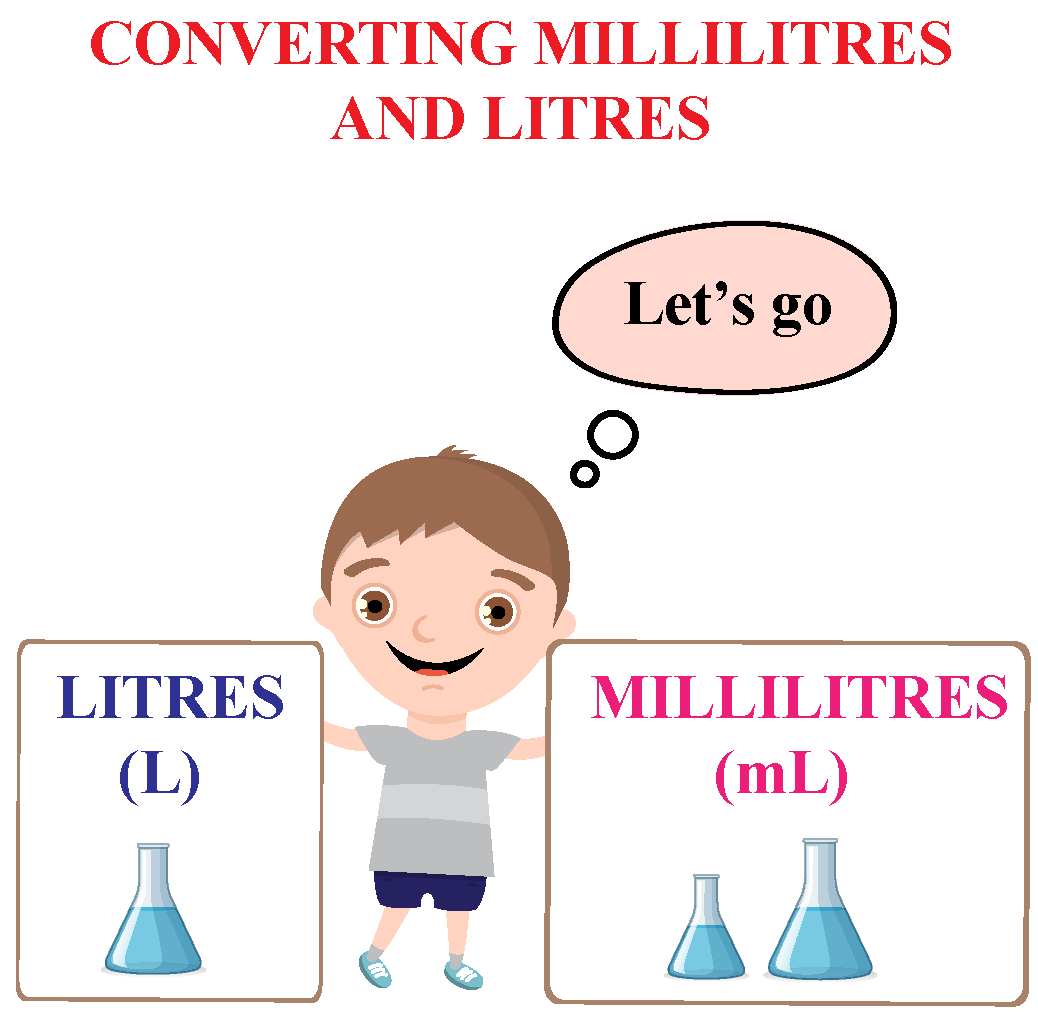## Lesson Plan

 1 Define Litres and Millilitres 2 How to Convert Litres to Millilitres? 3 Important Notes on Litres to millilitres 4 Challenging Questions on Litres to millilitres 5 Solved Examples on Litres to millilitres 6 Interactive Questions on Litres to millilitres

## Define Litres and Millilitres

### Litres

A litre is a basic metric unit that represents the volume.

It is used to measure liquids.

A litre is denoted with an abbreviation l or L and is measured as $$\ L^3$$.

A litre is slightly more than a quart.

The water pitcher below has exactly 1 L of water in it.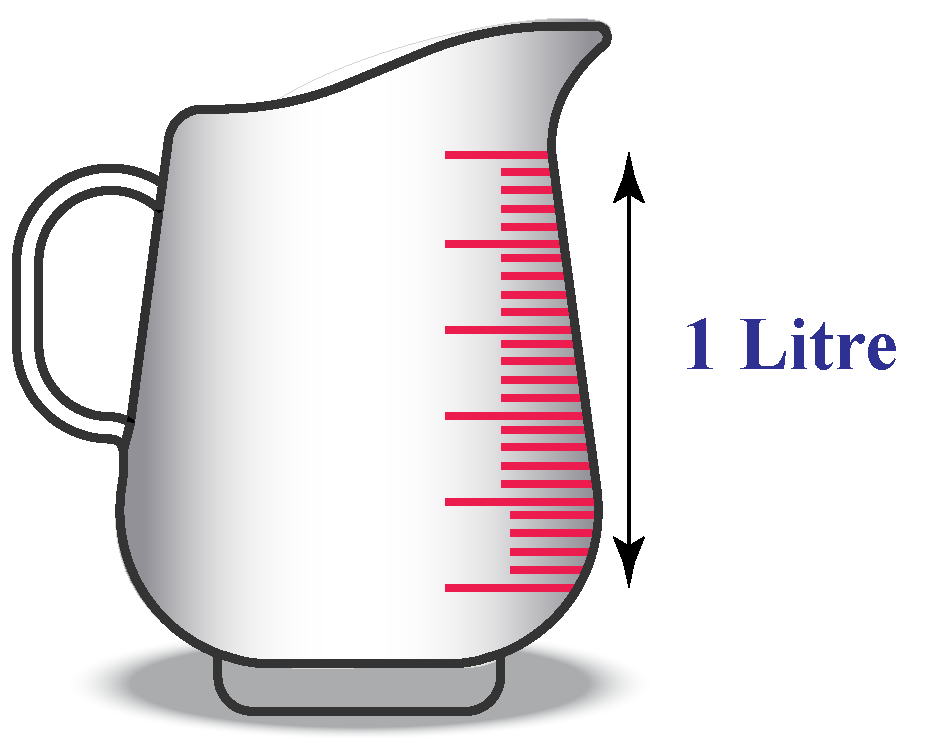### Millilitres

A millilitre is a smaller metric unit that represents the volume or capacity.

It is used to measure a smaller quantity of liquid.

A millilitre is denoted with an abbreviation ml or mL and is measured as $$\ mL^3$$.

The water pitcher below has exactly 1,000 mL of water in it.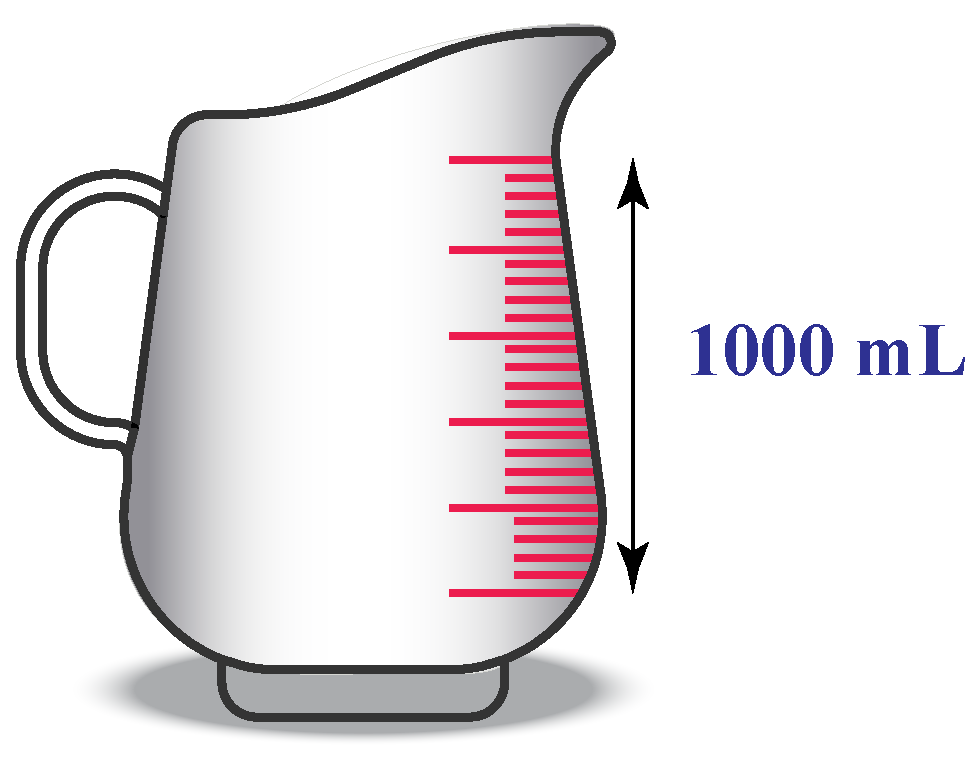A standard Pasteur pipette or an eyedropper holds 20 drops which is equal to 1 mL.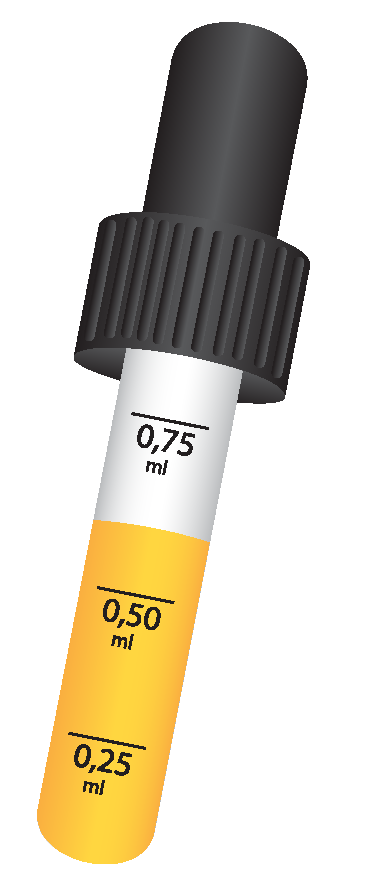These bottles can fill up to 200 ml of milk.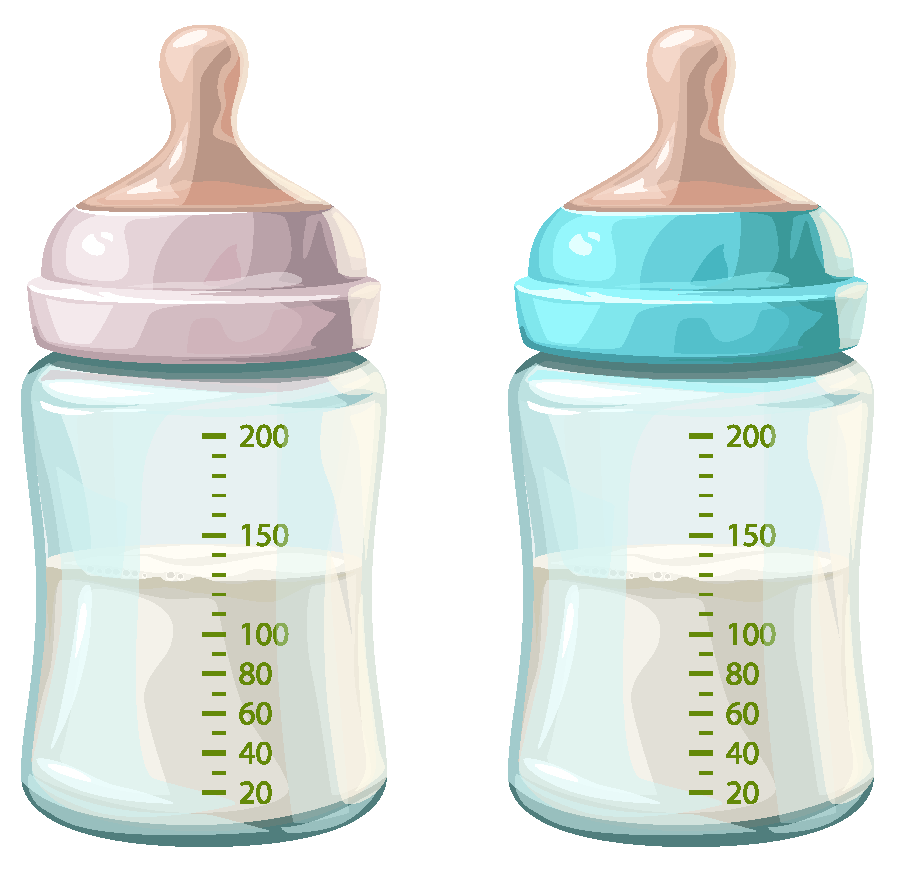## How to Convert Litres to Millilitres?

To convert litres to millilitres, you will be using two basic arithmetic operations i.e., division and multiplication.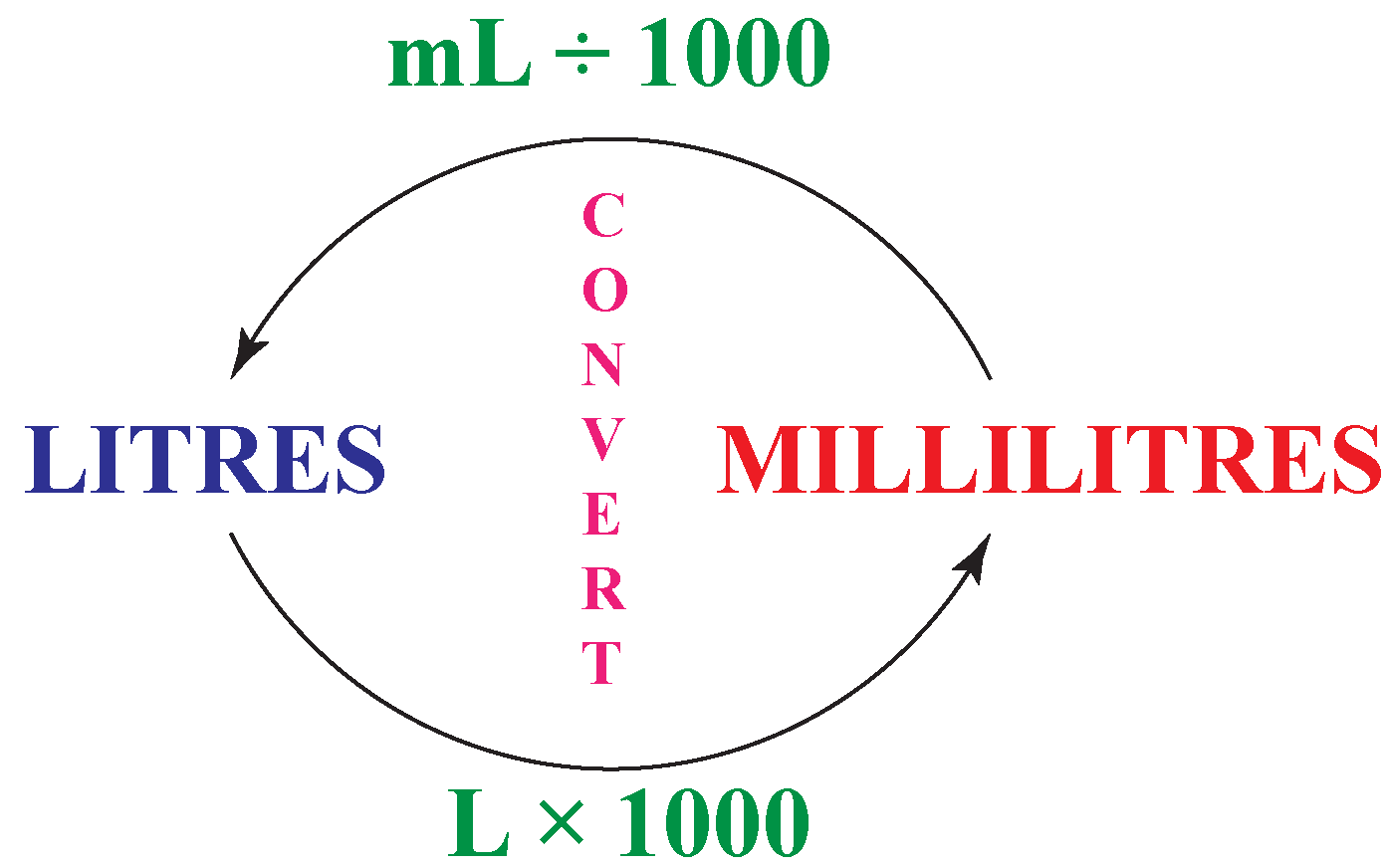Example 3

Ross bought 3 cans of orange juice of 1 L each.

He drank 1,500 mL of juice.

How much mL of juice is remaining in the cans?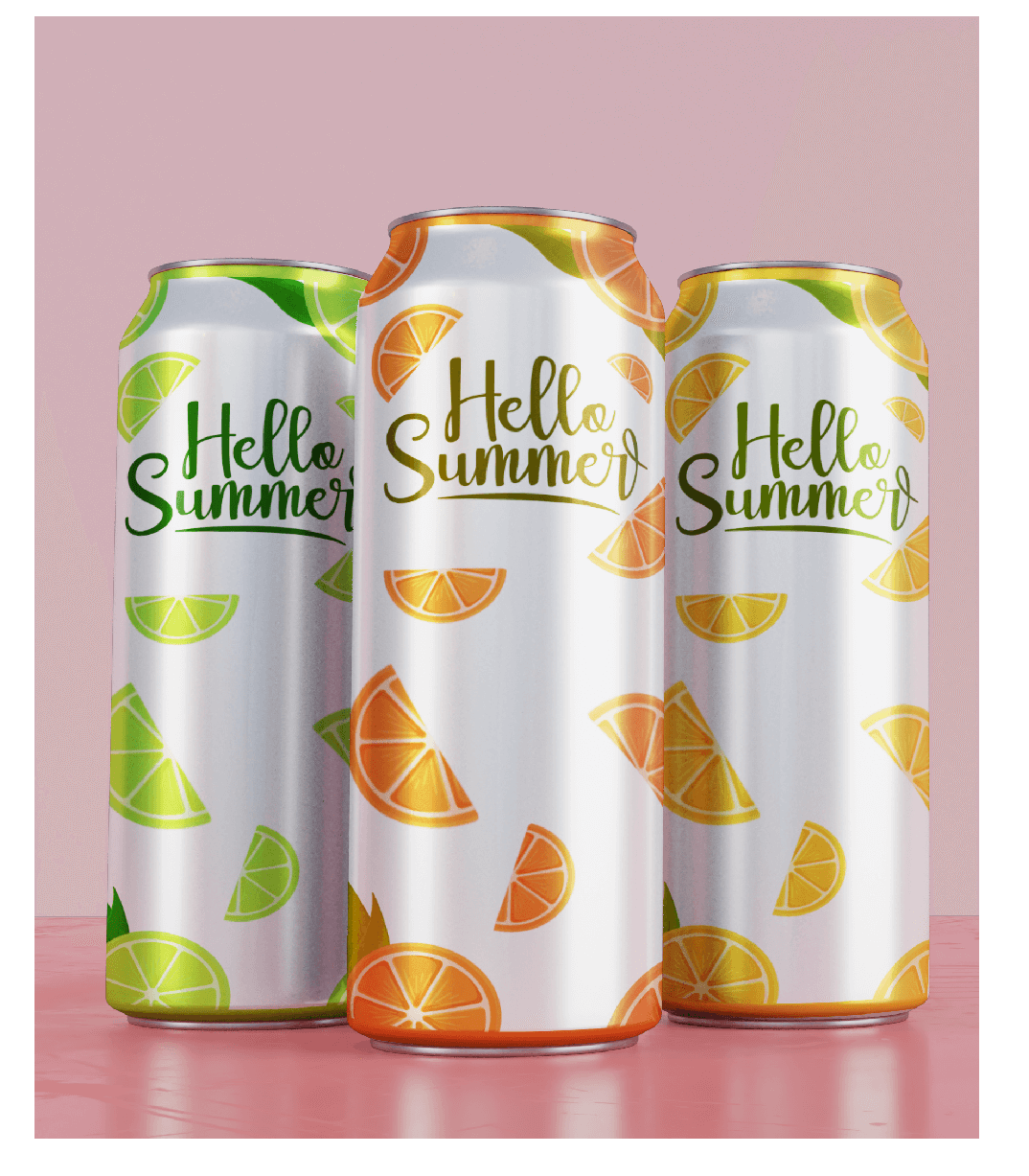Solution

\begin{align} 1\ can &= 1\ L\\3\ cans &= 3\ L\\ &= 3\times 1,000 = 3,000\ mL\\ &= 3,000\ mL - 1,500\ mL\\ &= 1,500\ mL\end{align}

 $$\therefore$$ 1,500 mL of juice is remaining.

## Interactive Questions

Here are a few activities for you to practice.

## Let's Summarize

We hope you enjoyed learning about litres to millilitres with the simulations and practice questions. Now you will be able to easily solve problems on converting litres to millilitres, how to calculate ml, ml to grams, and 1 litre to kg.

At Cuemath, our team of math experts is dedicated to making learning fun for our favourite readers, the students!

Through an interactive and engaging learning-teaching-learning approach, the teachers explore all angles of a topic.

Be it worksheets, online classes, doubt sessions, or any other form of relation, it’s the logical thinking and smart learning approach that we, at Cuemath, believe in.

## 1. Is 1 L the same as 750 mL?

No, $$\ 1\ L = 1,000\ mL.$$

$$\ 750\ mL = \frac{750}{1,000} = 0.75\ L$$

## 2. Is 1 L the same as 1000 mL?

Yes, $$\ 1\ L = 1,000\ mL$$

## 3. How many litres are in 80 mL of liquid?

$$\ 1\ L = 1,000\ mL$$

Thus, $$\ 80\ mL = \frac{80}{1,000} = 0.08\ L$$

More Important Topics
Numbers
Algebra
Geometry
Measurement
Money
Data
Trigonometry
Calculus